## Series Solutions: Airy's EquationThe general form of a homogeneous second order linear differential equation looks as follows:

y''+p(t) y'+q(t) y=0.

The series solutions method is used primarily, when the coefficients p(t) or q(t) are non-constant.

One of the easiest examples of such a case is Airy's Equation

y''-t y=0,

which is used in physics to model the defraction of light.

We want to find power series solutions for this second-order linear differential equation.

The generic form of a power series is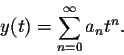We have to determine the right choice for the coefficients (an).

As in other techniques for solving differential equations, once we have a "guess" for the solutions, we plug it into the differential equation. Recall that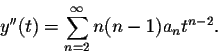Plugging this information into the differential equation we obtain: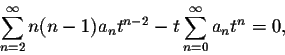or equivalently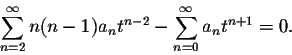Our next goal is to simplify this expression such that (basically) only one summation sign "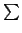" remains. The obstacle we encounter is that the powers of both sums are different, tn-2 for the first sum and tn+1 for the second sum. We make them the same by shifting the index of the first sum up by 2 units and the index of the second sum down by one unit to obtain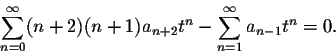Now we run into the next problem: the second sum starts at n=1, while the first sum has one more term and starts at n=0. We split off the 0th term of the first sum: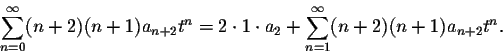Now we can combine the two sums as follows: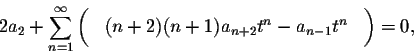and factor out tn: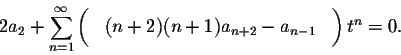The power series on the left is identically equal to zero, consequently all of its coefficients are equal to 0: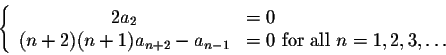We can slightly rewrite as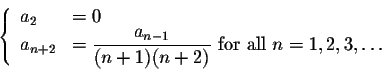These equations are known as the "recurrence relations" of the differential equations. The recurrence relations permit us to compute all coefficients in terms of a0 and a1.

We already know from the 0th recurrence relation that a2=0. Let's compute a3 by reading off the recurrence relation for n=1: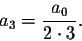Let us continue: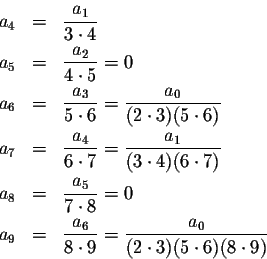The hardest part, as usual, is to recognize the patterns evolving; in this case we have to consider three cases:

1. All the terms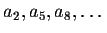are equal to zero. We can write this in compact form as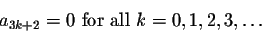2. All the terms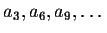are multiples of a0. We can be more precise: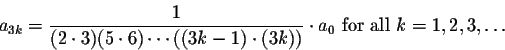(Plug in k=1,2,3,4 to check that this works!)

3. All the terms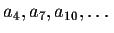are multiples of a1. We can be more precise: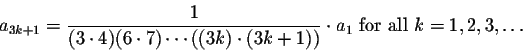(Plug in k=1,2,3,4 to check that this works!)

Thus the general form of the solutions to Airy's Equation is given by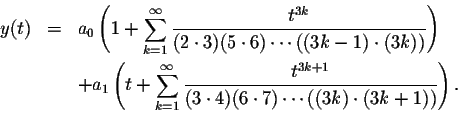Note that, as always, y(0)=a0 and y'(0)=a1. Thus it is trivial to determine a0 and a1 when you want to solve an initial value problem.

In particular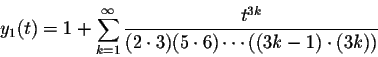and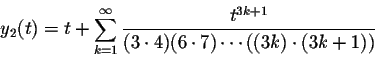form a fundamental system of solutions for Airy's Differential Equation.

Below you see a picture of these two solutions. Note that for negative t, the solutions behave somewhat like the oscillating solutions of y''+y=0, while for positive t, they behave somewhat like the exponential solutions of the differential equation y''-y=0.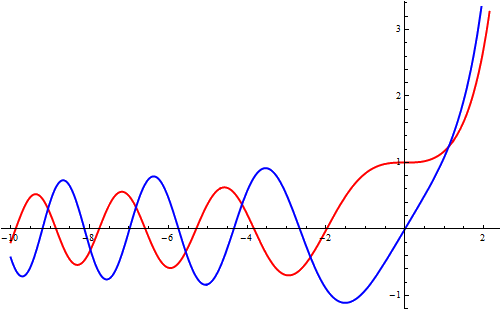In the next section we will investigate what one can say about the radius of convergence of power series solutions.[Back] [Next]
[Algebra] [Trigonometry] [Complex Variables]
[Calculus] [Differential Equations] [Matrix Algebra]S.O.S. MATHematics home page

Do you need more help? Please post your question on our S.O.S. Mathematics CyberBoard.Helmut Knaust
1998-07-03